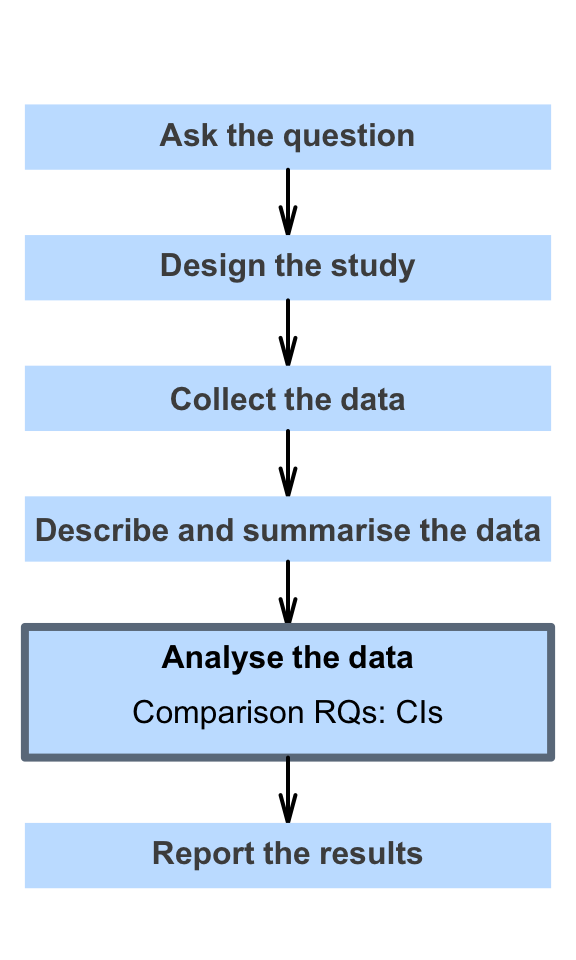# 19 Introducing confidence intervals

One type of research question, is the estimation-type RQ, when the precision of a statistic is of interest. In this Part, answering estimation-type RQs is discussed for:

• Descriptive RQs: Chaps. 20 to 23;
• Relational or interventional RQs with a comparison: Chaps. 24 and 25).

Answering estimation-type RQs for relational or interventional RQs with a connection is explored later (Chaps. 34 and 35).

The precision of statistics influences decision-type RQ too: when statistics precisely estimate parameters, making decisions about parameters is easier.

The previous chapters, where tools for describing sampling variation were introduced, are used in this part, to understand the precision of statistics.